﻿ 船体阻力数值预报研究及黏性流场计算
 舰船科学技术2020, Vol. 42Issue (1): 17-22PDF

1. 渤海船舶职业学院 船舶工程学院，辽宁 葫芦岛 125001;
2. 上海海事大学 商船学院，上海 201306;
3. 哈尔滨工程大学 动力与能源工程学院，黑龙江 哈尔滨 150001;
4. 常熟理工学院 汽车学院，江苏 常熟 215500

Research on resistance prediction and calculation of viscous flow field
WANG Ying-di1, CHEN Yan-zhen2, ZHOU Wei-jian3, ZHANG Sheng-long4
1. Bohai Shipbuilding Vocational College, Huludao 125105, China;
2. Shanghai Maritime University, Merchant Maritime College, Shanghai 201306, China;
3. Harbin Engineering University, College of Power and Energy Engineering, Haerbin 150001, China;
4. Changshu Institute of Technology, Automotive Engineering, Changshu 215500, China
Abstract: In this paper, the accuracy of resistance prediction was studied by using the RANS equations based on the SIMPLE algorithm with KCS. The free surface was simulated with volume of fluid (VOF) method. The whole computational domain was divided with trimmed mesh. Taking the MDOF model as an example, firstly, the influence of boundary layer and turbulence coefficients were analyzed, which provided the basis for accurate prediction of hull resistance. In the process of numerical simulation, the high-quality mesh and the high-precision numerical method are the key to CFD simulation. The paper established the optimization system of turbulence coefficients based on non-linear programming by quadratic lagrangian (NLPQL) algorithm to find the optimal solution parameters. Then, the total resistance, the pressure on wet surface of hull and the flow field under different Fr were calculated in the most suitable grids and turbulence coefficients. The differences between captive model and MDOF model in resistance prediction and flow field were discussed. Then the numerical results were compared with the experimental data. This study can give a reference for the future research in resistance prediction for the ship hull.
Key words: SIMPLE     KCS     resistance prediction     NLPQL
0 引　言

1 CFD数学模型建立

2 几何模型及网格划分表 1 KCS船型主尺度 Tab.1 Principal dimensions of KCS ship types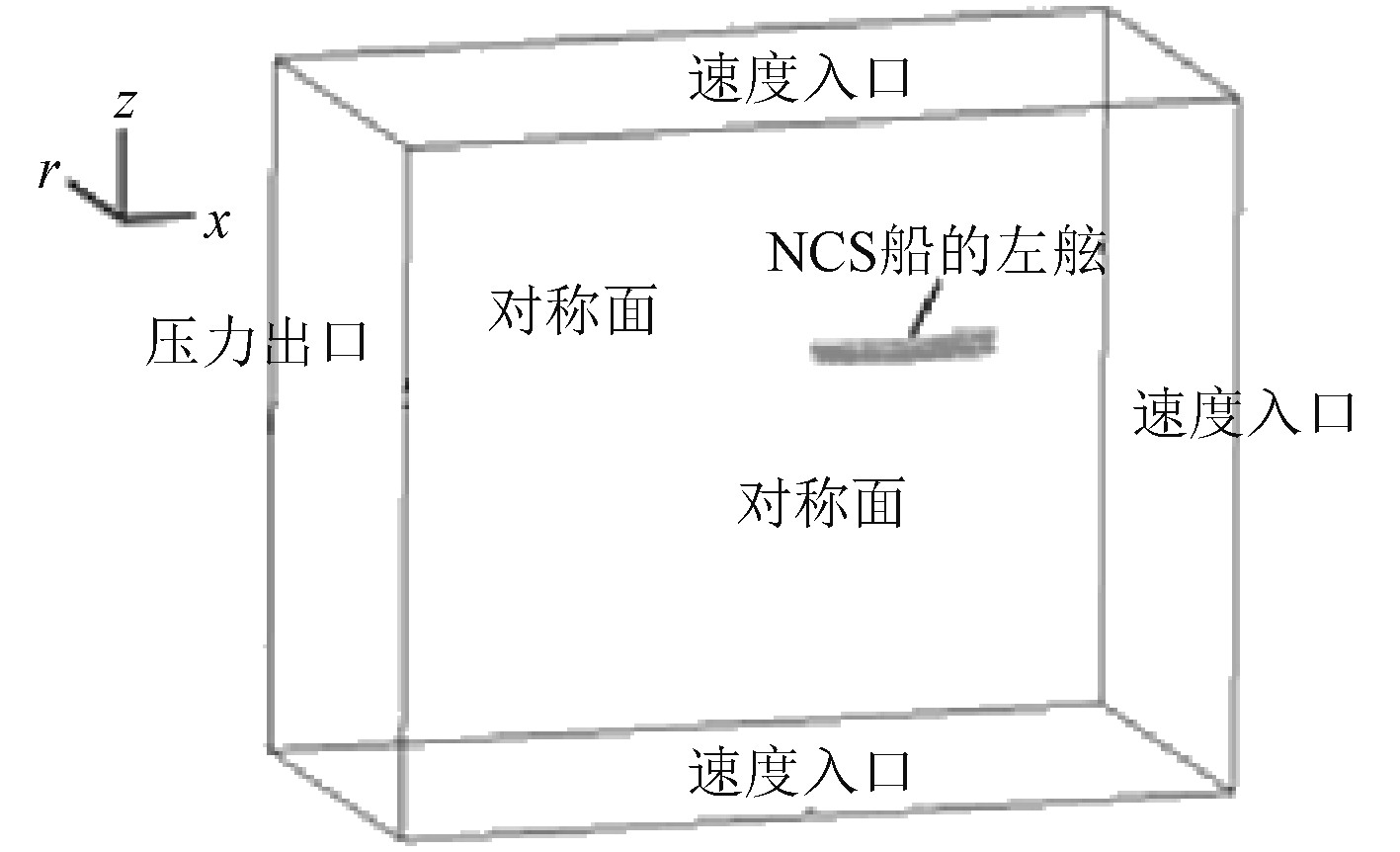图 1 边界条件设置 Fig. 1 Boundary conditions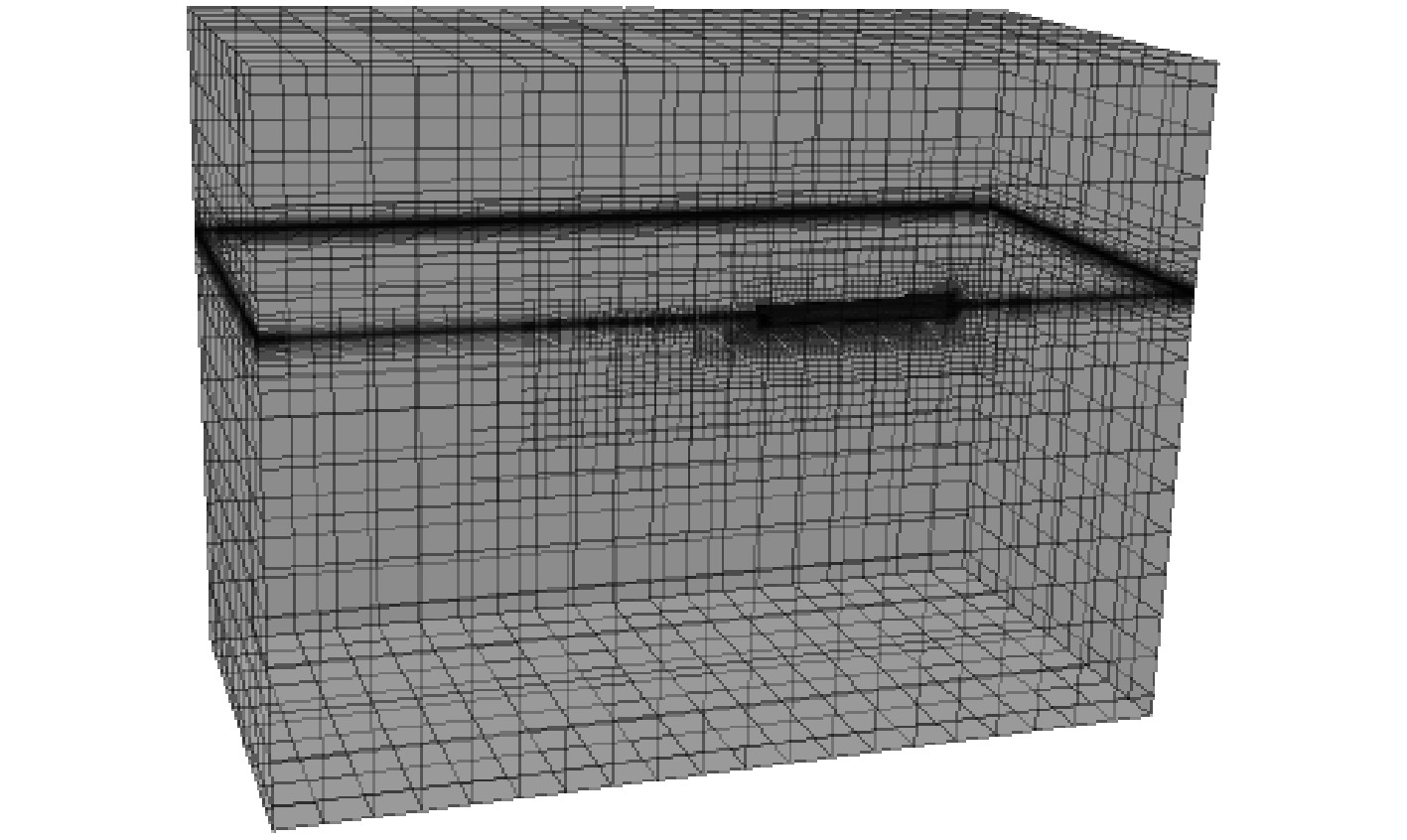图 2 整个计算域网格划分 Fig. 2 Mesh on the computational domain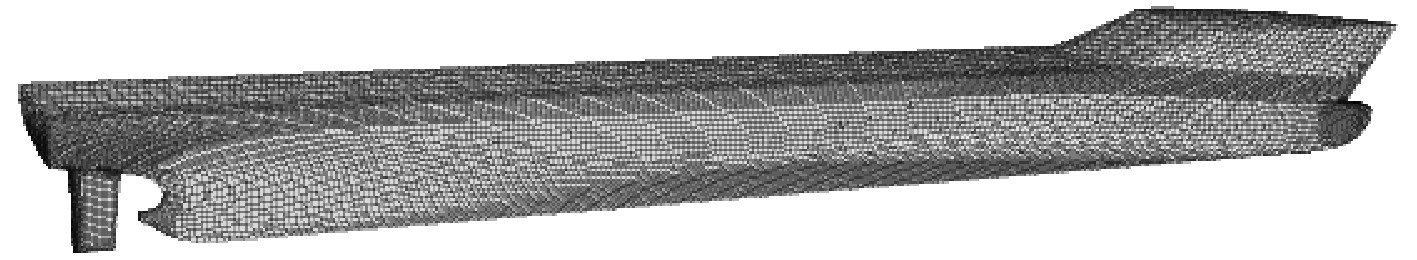图 3 船体及舵表面网格划分 Fig. 3 Mesh on the hull and rudder surface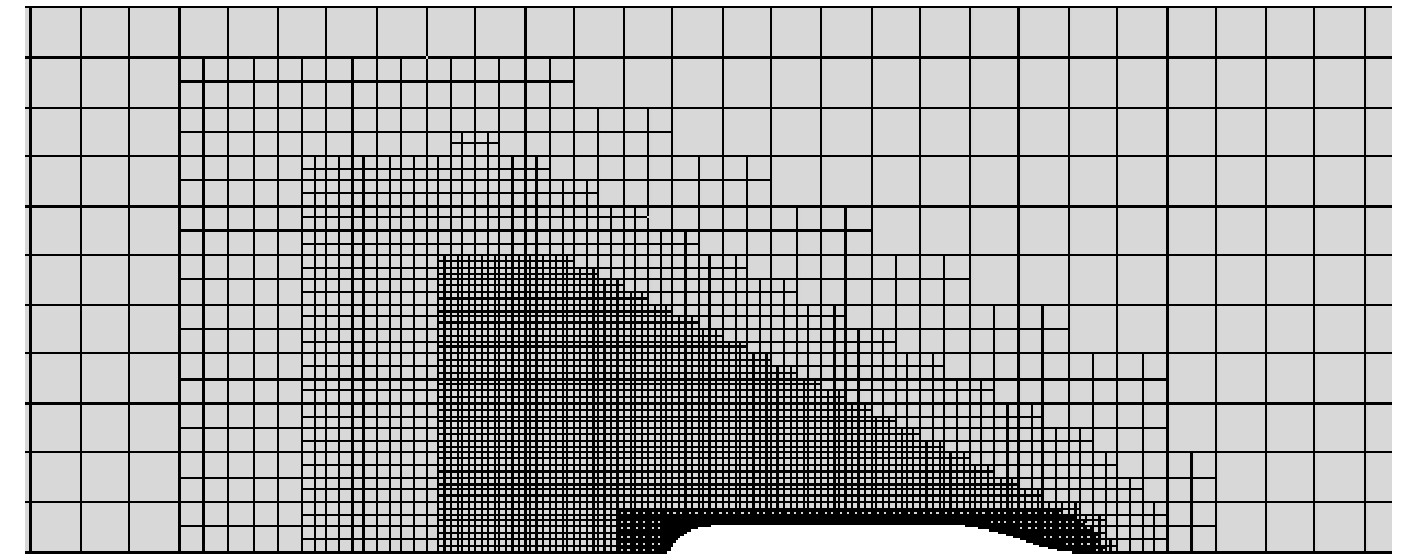图 4 自由液面网格划分 Fig. 4 Mesh on the free surface
3 阻力预报流程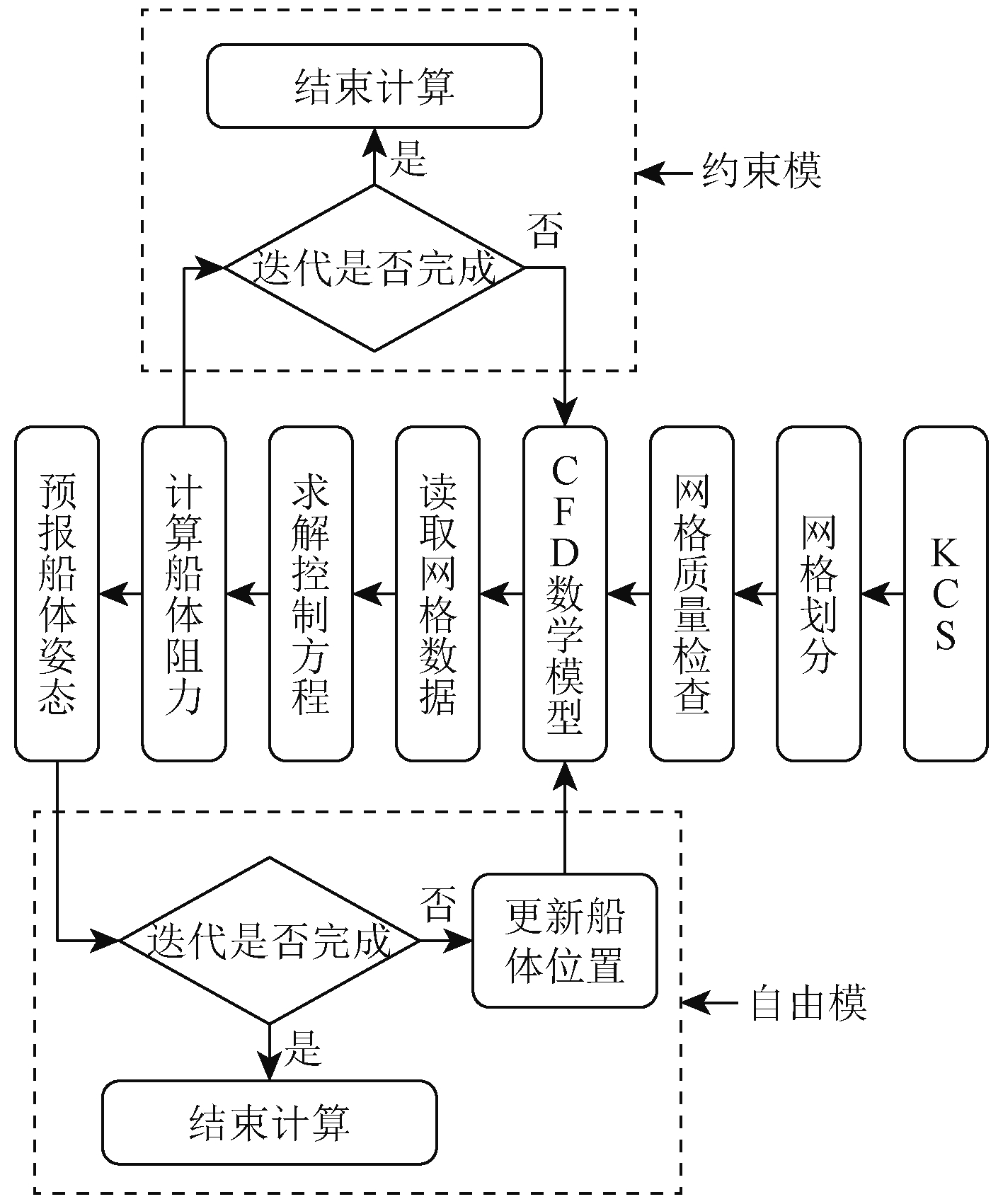图 5 计算流程图 Fig. 5 Computational flow chart
4 CFD模型参数影响分析 4.1 边界层对阻力的影响

 ${{{y}}^ + }=0.172(\frac{y}{{{L_{{\rm{pp}}}}}}){{Re} ^{0.9}}{\text{。}}$ (1)表 2 首层边界层厚度与阻力之间的关系 Tab.2 The relation between the thickness of the first boundary layer and the resistance表 4 边界层数与阻力之间的关系 Tab.4 The relationship between the number of boundary layers and resistance表 3 网格增长因子与阻力之间的关系 Tab.3 The relationship between grid growth factor and resistance
4.2 湍流系数对阻力的影响表 5 Realizable k-ε湍流模型系数 Tab.5 Realizable k-ε turbulence model coefficients表 6 ${C_\mu }$ 与阻力之间关系 Tab.6 The relationship between ${C_\mu }$ and resistance表 10 ${\alpha _{\varepsilon} }$ 与阻力之间关系 Tab.10 The relationship between ${\alpha _{\varepsilon} }$ and resistance表 7 ${C_{1\varepsilon }}$ 与阻力之间关系 Tab.7 The relationship between ${C_{1\varepsilon }}$ and resistance表 8 ${C_{2\varepsilon }}$ 与阻力之间关系 Tab.8 The relationship between ${C_{2\varepsilon }}$ and resistance表 9 ${\alpha _k}$ 与阻力之间关系 Tab.9 The relationship between ${\alpha _k}$ and resistance

5 模型参数优化及多航速阻力预报 5.1 模型参数优化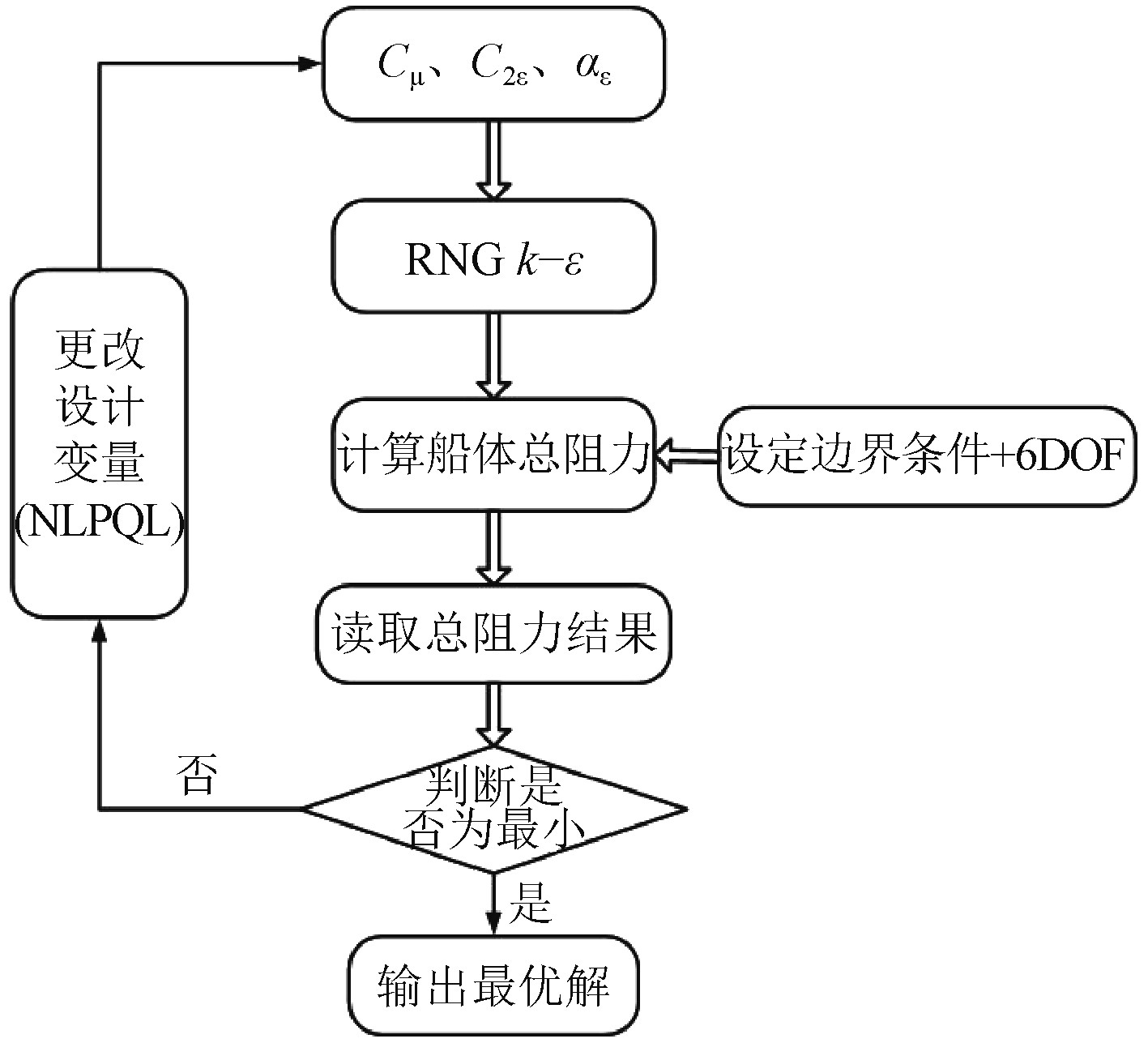图 6 优化流程 Fig. 6 Optimization process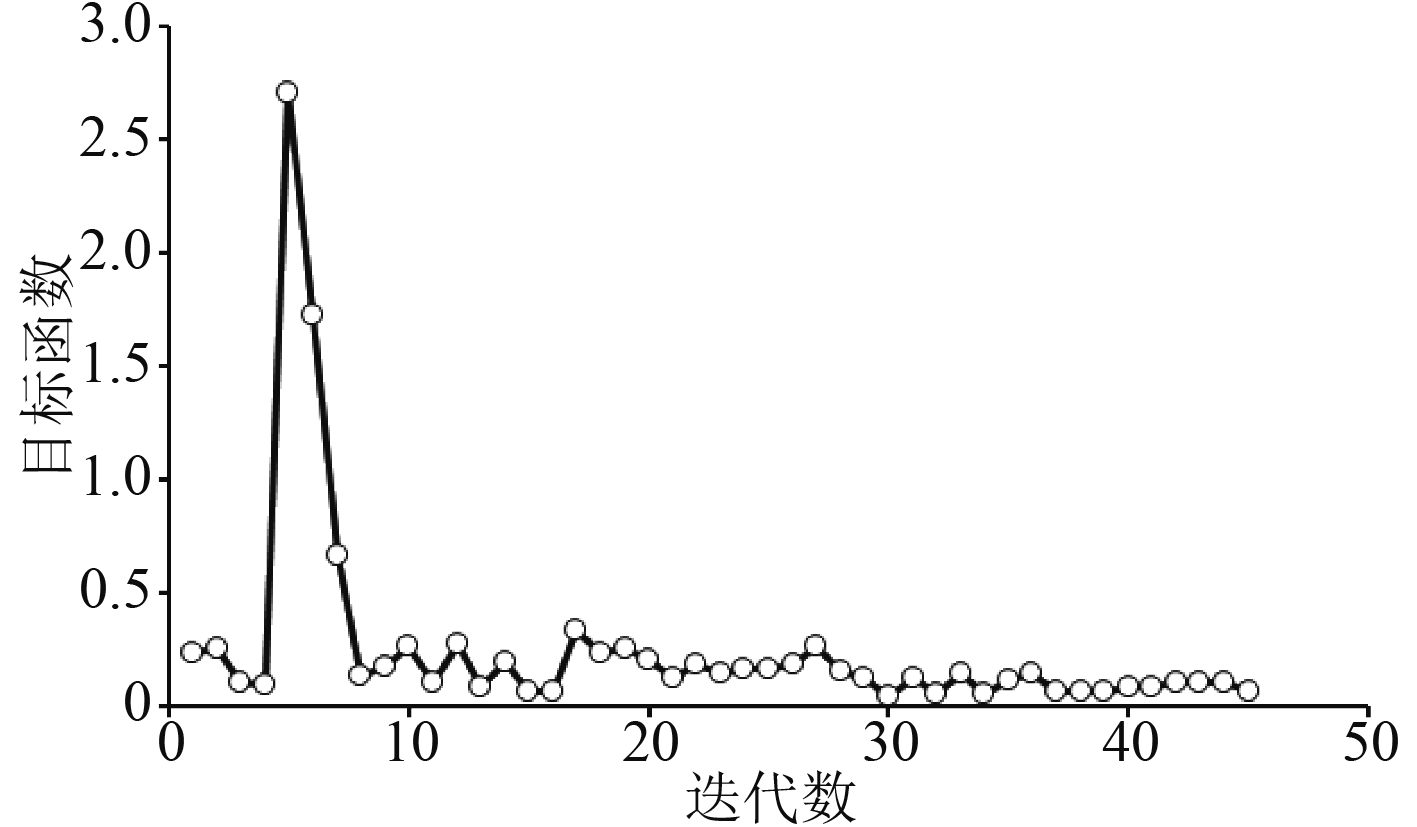图 7 序列二次规划算法优化过程 Fig. 7 The optimization process for the NLPQL algorithm表 11 模型系数选取 Tab.11 The selection of Realizable k-ε turbulence model coefficients
5.2 多航速阻力预报表 12 总阻力计算结果与实验值对比 Tab.12 The comparison of the Ct calculated by CFD and EFD methods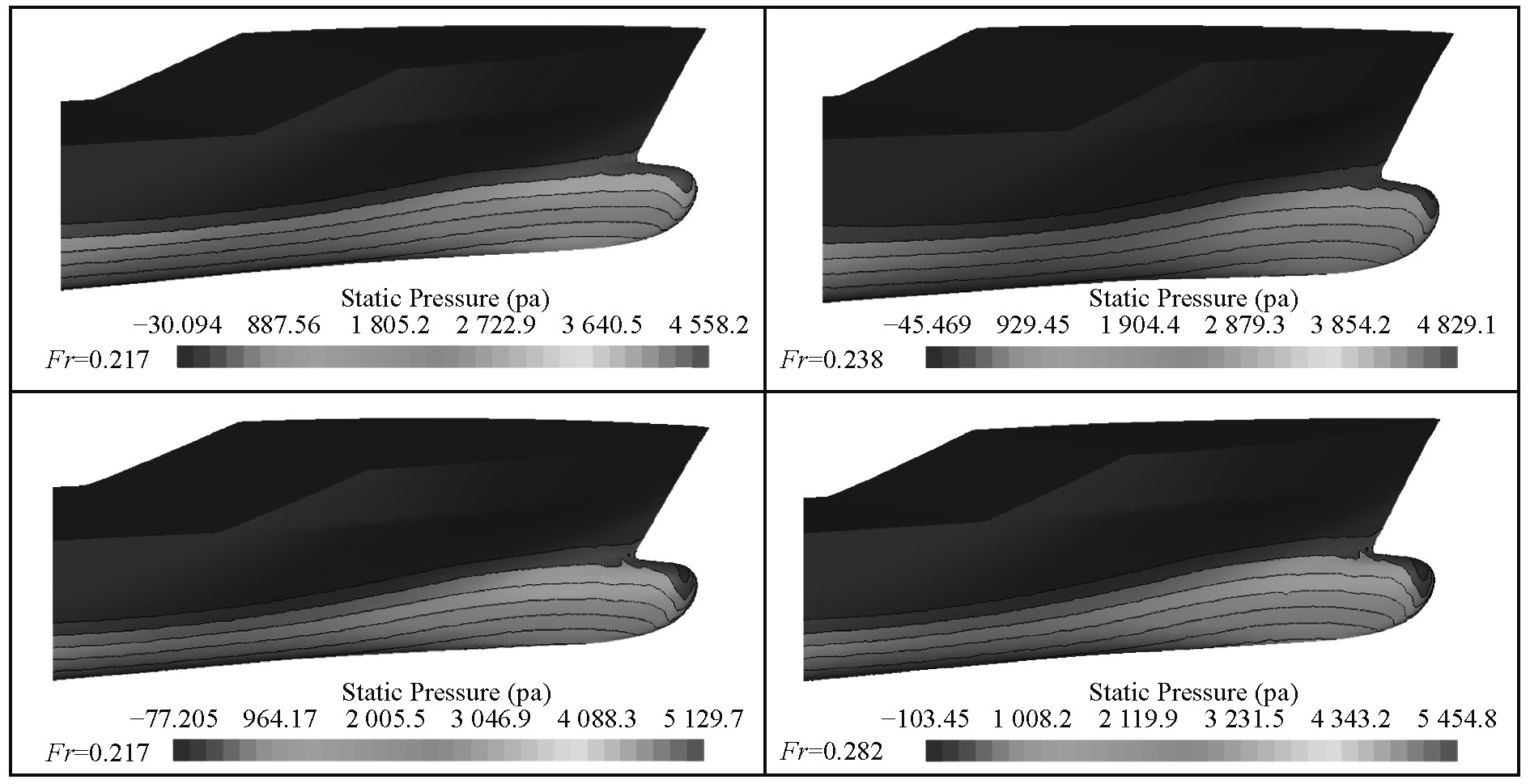图 8 船首表面压力分布（约束模—推荐系数） Fig. 8 Static pressure distribution on the bow section（restraint mode-recommendation coefficients）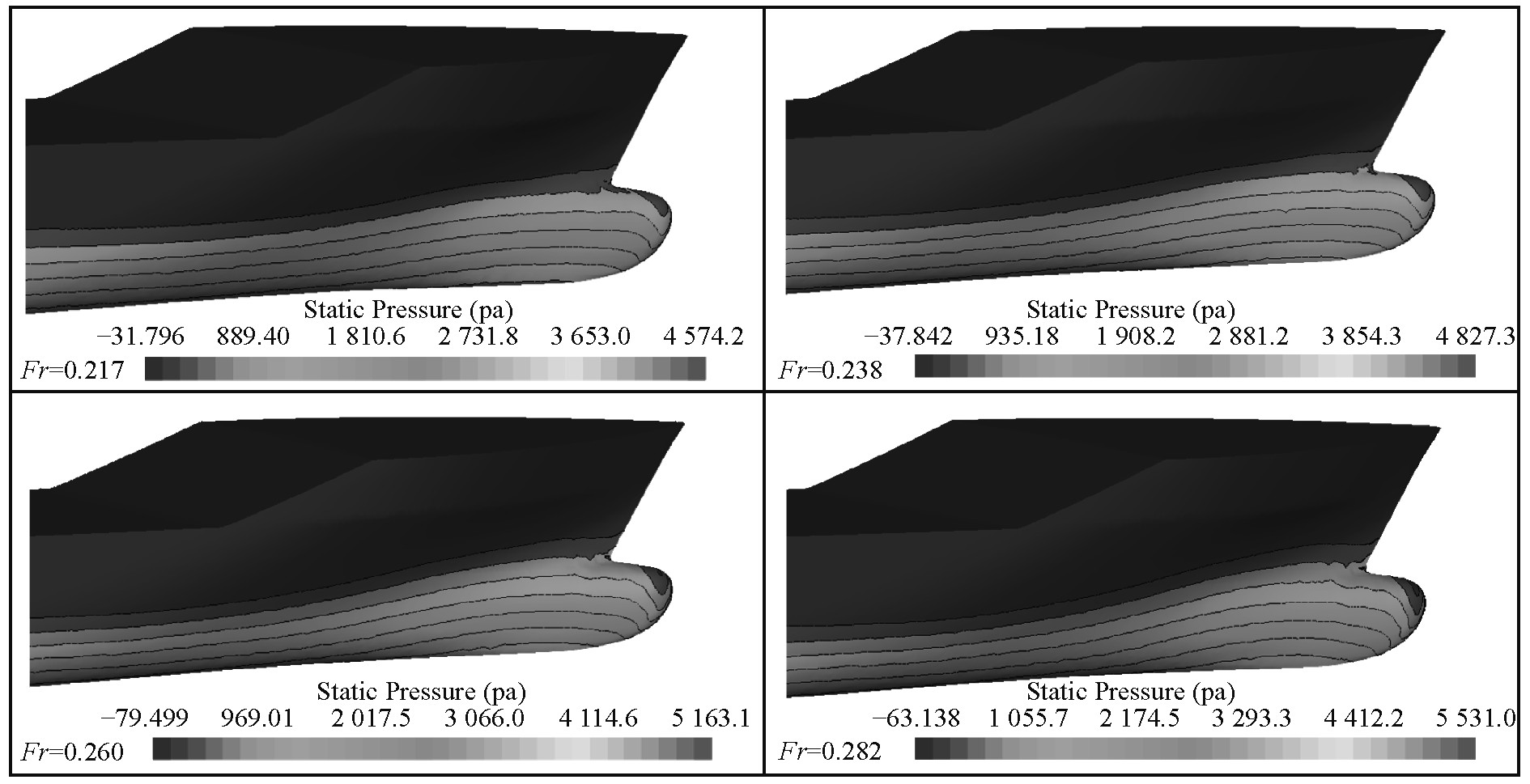图 9 船首表面压力分布（自由模—推荐系数） Fig. 9 Static pressure distribution on the bow section（free mode-recommended coefficients）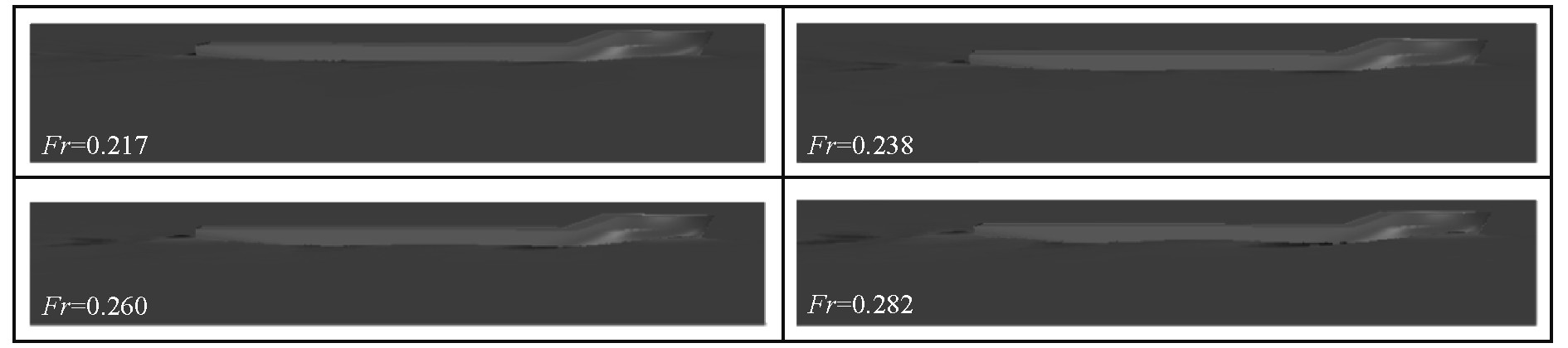图 10 稳定时刻船体姿态（自由模—最优系数） Fig. 10 Ship attitude at stable time（free mode-optimal coefficients）表 13 纵倾值和垂荡变化值与文献值对比 Tab.13 The comparison of pitch and heave values obtained by CFD method and previous literature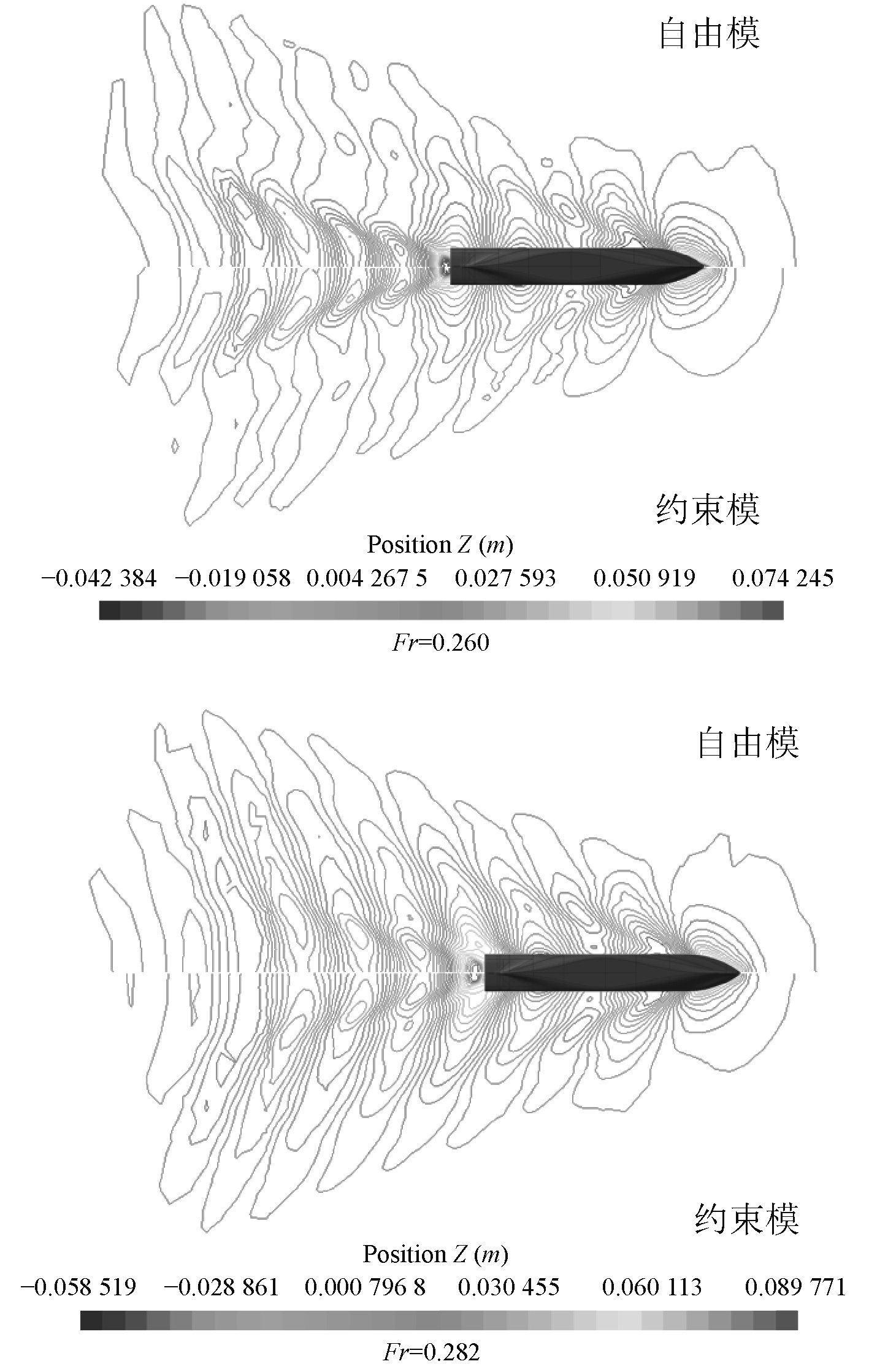图 11 推荐系数波形等高线对比图 Fig. 11 The comparison of the waveform contour obtained by recommended coefficients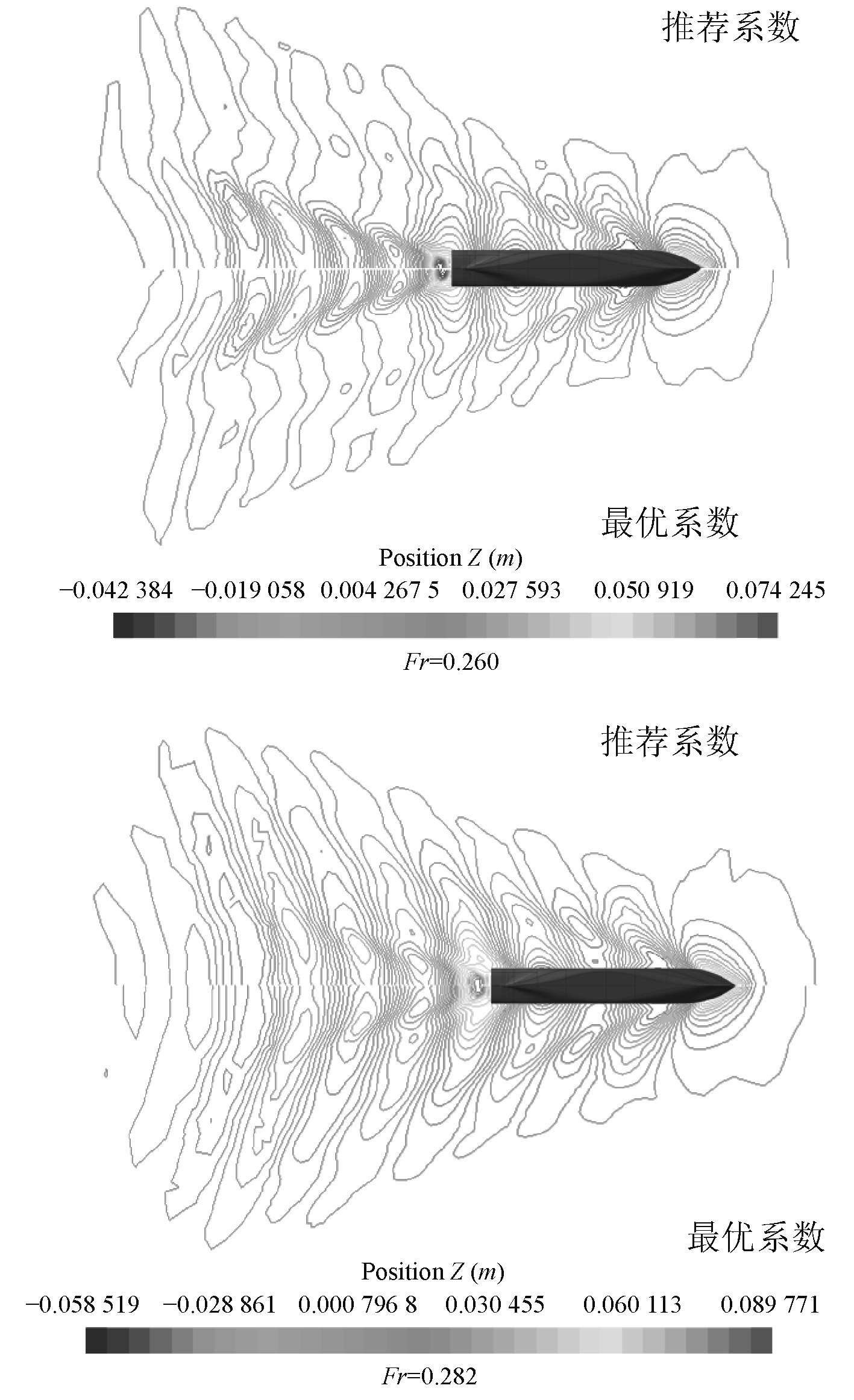图 12 自由模波形等高线对比图 Fig. 12 The comparison of the waveform contour obtained by free-mode
6 结　语

1）船体附近边界层划分方式对阻力预报有较大的影响。随着首层边界层厚度的增加，阻力也随之增加；随着边界层数的增加，阻力减小；而网格增长因子与阻力之间没有明显的线性关系。

2） ${C_{2\varepsilon }}$ ${\alpha _{\varepsilon} }$ 对阻力的影响较大，而 ${C_{1\varepsilon }}$ ${\alpha _k}$ 的变化对阻力影响较小。随着 ${C_\mu }$ ${\alpha _k}$ 的增加，阻力减小；随着 ${C_{2\varepsilon }}$ ${\alpha _{\varepsilon} }$ 的增加，阻力增加；而 ${C_{1\varepsilon }}$ 与阻力之间没有明显的线性关系，但当 ${C_{1\varepsilon }}$ >1.42时，阻力随着 ${C_{1\varepsilon }}$ 的增加而减小。其次，构建了基于NLPQL法的优化系统，得到了适合KCS船体阻力预报的湍流系数： ${C_\mu }$ =0.09， ${C_{1\varepsilon }}$ =1.44， ${C_{2\varepsilon }}$ =1.950 5， ${\alpha _k}$ =1.0， ${\alpha _{\varepsilon} }$ =1.187 5。

3）自由模在船体阻力预报精确度上优于约束模，误差只有0.95%。采用最优湍流系数对船体阻力进行预报时，除了Fr=0.282以外，其他航速阻力计算精确度均高于推荐湍流系数。

  CAMPANA E F, PERI D. Shape optimization in ship hydrodynamics using computational fluid dynamics[J]. Comput. Methods Appl. Mech. Engrg, 2006, 196: 634-651. DOI:10.1016/j.cma.2006.06.003  PERI D, ROSSETTI M, CAMPANA E F. Multidisciplinary design optimization of a naval surface combatant[J]. Journal of Ship Rearch, 2003, 41(1): 1-12.  TAHARA Y, STERN F, HIMENO Y. Comuputational fluid dynamics-based optimization of a surface combatant[J]. Journal of Ship Rearch, 2004, 28(4): 273-287.  DUMMETT R. The use of airboats in ice and water rescue emergencies-equipment that can minimize personnel risk and substantially reduce the time required for a life-saving rescue[J]. Fire Engineering, 2004, 157(3): 113-126.  YEK P N Y. Static stability study of a shallow vee hull airboat for sarawak riverine application[D]. University Malaysia Sarawak, UNIMAS, 2014.  PHAN Q T, NGO K H, PHAM M V. Numerical simulation of floating airboat: estimation of hydrodynamic forces[J]. International Journal of Mechanical Engineering and Applications, 2015, 13(3): 41-46.  倪崇本. 基于CFD的船舶阻力性能综合研究[D]. 上海: 上海交通大学, 2011.  张艳, 阙晓辉. 基于CFD的船舶船体总阻力预报方法[J]. 舰船科学技术, 2015, 37(3): 139-142. DOI:10.3404/j.issn.1672-7649.2015.03.030  孔金平, 吴波涛, 孔令志. 基于CFD的船舶双螺旋桨水动力性能分析[J]. 舰船科学技术, 2019, 41(3): 37-41.  HIRT, C W & NICHOLS, B D. Volume of fluid method for the dynamics of free boundaries[J]. Comp. Phys, 1981, 39: 20-225.  徐晓宏, 赵万忠, 王春燕, 等. 基于NLPQL算法的电动轮汽车差速助力转向参数优化[J]. 中南大学学报(自然科学版), 2012, 43(9): 3431-3436.  郭春雨, 王恋舟, 赵庆新, 等. 一种计及姿态变化的船舶阻力预报方法[J]. 船舶工程, 2015, 37(01): 31-34.